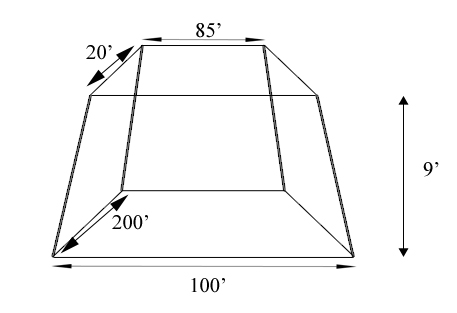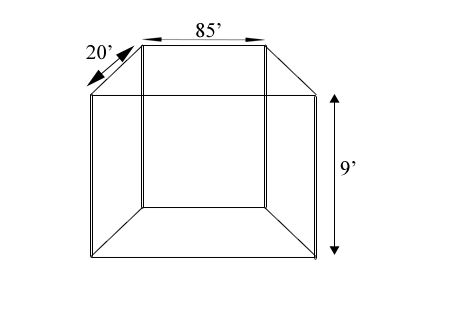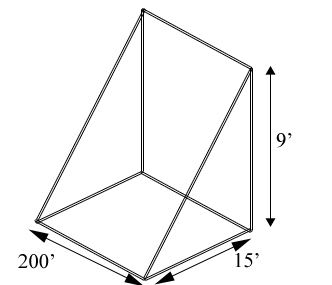SEARCH HOMEMath Central Quandaries & QueriesQuestion from ron, a student: hi i have a berm 100'w x200'L at the base and 9'tall the top is 85'L x 20'w i would to find out how many cubic yards are in the pile and the formulaHi Ron,

The object you described is an irregular shape so it is best to divide it into "regular" shapes.Since the sides are slanted, it will be easiest to divide this irregular shape into one rectangle that is 20'x85'x9'and four triangular prisms that are 200'x15'x9'. (I got the base of 15' from 100-85=15)You can now find the volume of each shape using our volume calculator and add them together.

Hope this helps,

JaniceMath Central is supported by the University of Regina and The Pacific Institute for the Mathematical Sciences.